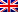#[EasyCTF 2018] [Reverse 150 – Soupstitution Cipher] Write Up

## Description

For this challenge, we need to reverse a briefly obfuscated python script to find an input value that will validate some conditions to get the flag.

```#!/usr/bin/env python3

from binascii import unhexlify as sOup
from operator import attrgetter as souP

ME_FLAGE = 'censored'

SoUp = input
soUP = hex
sOUp = print
sOuP = ord
SOuP = open

def SoUP(sOUP):
soup = 0
while sOUP != 0:
soup = (soup * 10) + (sOUP % 10)
sOUP //= 10
return soup

def SOup(sOUP):
soup = 0
for soUp in sOUP:
soup *= 10
soup += sOuP(soUp) - sOuP('0')
return soup

def SOUP():
Soup = SoUp()[:7]
print(Soup)
if not souP('isdigit')(Soup)():
sOUp("that's not a number lol")
return

soup = SoUP(SOup(Soup))
SouP = souP('zfill')(soUP(soup)[2:])(8)[-8:]
if sOup(SouP) == souP('encode')('s0up')():
sOUp("oh yay it's a flag!", ME_FLAGE)
else:
sOUp('oh noes rip u')

if __name__ == '__main__':
SOUP()

```

#[Trend Micro 2016] [Misc 100 – PCAP] Write Up

## Description

Category: Misc(iot and network)
Points: 100